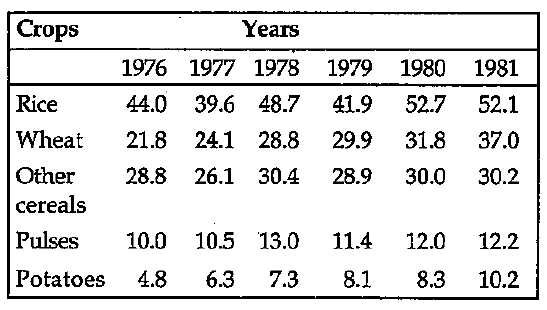# Data Interpretation - Tabular Data

>>>>>>>>Tabular Data

Instructions:

Study the following table carefully and answer the question given below it:• A

Wheat• B

Pulses• C

Rice• D

None of these• Option : C
• Explanation : In case of rice; the production in 1980 increases by 10.8 lakh tonnes as compared to 1979

Instructions:

Study the following table carefully and answer the question given below it:

• A

1979• B

1978• C

1977• D

None of these• Option : C
• Explanation : The production of rice and wheat together in 1976, 1977, 1978, 1979, 1980 and 1981 was 65.8, 63.7, 77.5, 71.8, 84.5 and 89.1 lakhs tonnes respectively

Instructions:

Study the following table carefully and answer the question given below it:

• A

Rice• B

Wheat• C

Pulses• D

None of theseInstructions:

Study the following table carefully and answer the question given below it:

• A

Wheat• B

Other cereals• C

Potatoes• D

None of these• Option : A
• Explanation : The percentage increase of production in 1981 over that in 1976 in case of:

Rice = [(52.1-44)*100]  x100 = 18.41%

Wheat = [(37-21.8)*100]  x100 = 69.72%

Pulses = [(12.2-10)*100]  x100 = 22%

Potatoes = [(10.2-4.8)*100]  x100 = 112.5%

• A• BRelated Quiz.
Tabular Data